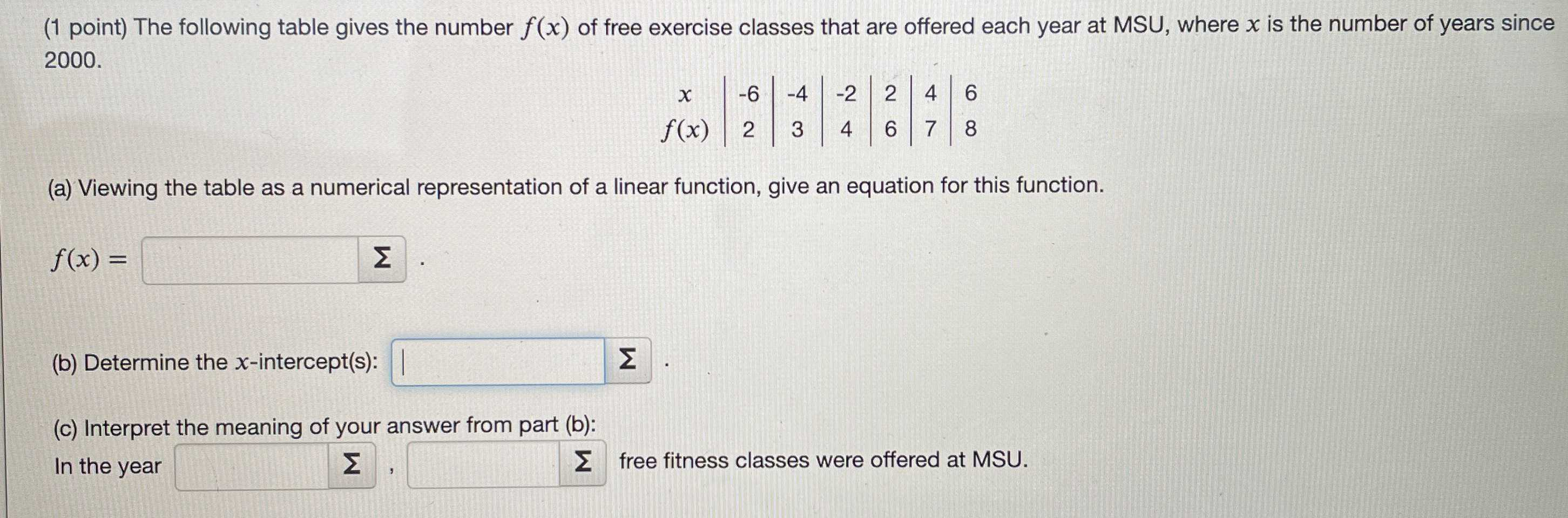### ¿Todavía tienes preguntas de matemáticas?

Pregunte a nuestros tutores expertos
Algebra
Pregunta(1 point) The following table gives the number $$f ( x )$$ of free exercise classes that are offered each year at MSU, where $$x$$ is the number of years since $$2000 .$$

(a) Viewing the table as a numerical representation of a linear function, give an equation for this function.

$$f ( x ) = \square \quad$$

(b) Determine the $$x$$ -intercept(s): $$\square$$

In the year $$\square$$,$$\square$$ free fitness classes were offered at MSU.